# Shape formed by rotating a circular arc about an axis defined by its two end points

murrdpirate0
A 180 degree circular arc (i.e. a half sphere) is obvious: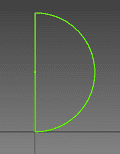When you rotate this about its two end points, you get a sphere.

What about for something less than 180 degrees (e.g. 90)?: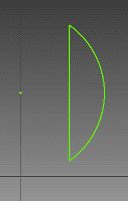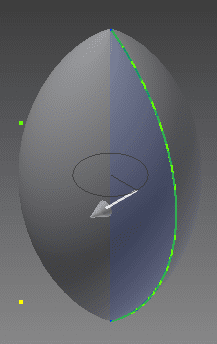I believe this forms an ellipsoid, with coefficients a and b being equal, with respect to wikipedia's entry on ellipsoid: http://en.wikipedia.org/wiki/Ellipsoid. Can anyone confirm that is correct?

I have no idea what it would be for something greater than 180 degrees: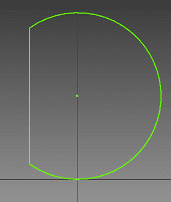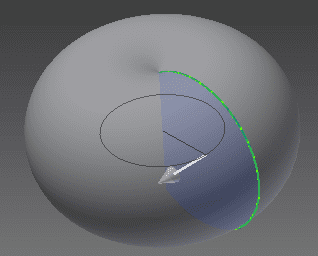Its cross section would look like a butterfly. I have no idea what the 3D shape would be called. Anyone have any idea? I'm especially interested in obtaining an equation that would describe its surface.

Last edited:

## Answers and Replies

Staff Emeritus
Homework Helper

A 180 degree circular arc (i.e. a half sphere) is obvious:When you rotate this about its two end points, you get a sphere.

What about for something less than 180 degrees (e.g. 90)?: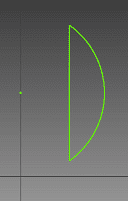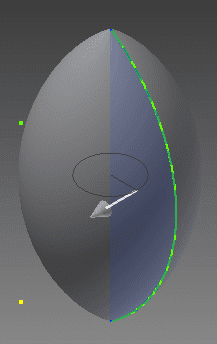I believe this forms an ellipsoid, with coefficients a and b being equal, with respect to wikipedia's entry on ellipsoid: http://en.wikipedia.org/wiki/Ellipsoid. Can anyone confirm that is correct?

It cannot be an ellipsoid. The reason is that an ellipsoid is smooth everywhere, while your surface has "cusps" on the bottom and on the top.

I have no idea what it would be for something greater than 180 degrees: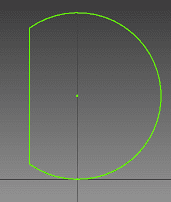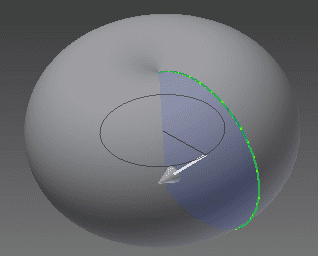Its cross section would look like a butterfly. I have no idea what the 3D shape would be called. Anyone have any idea? I'm especially interested in obtaining an equation that would describe its surface.

It looks like a spindle torus: http://en.wikipedia.org/wiki/Spindle_torus

murrdpirate0

I was just looking at the spindle torus and I agree, that appears right. It would be preferable if I could have the math only describe the outer shell, but maybe that's not practical.

Good point about the other shape not being an ellipsoid. I don't think I would have noticed that. I guess it's actually the inner surface of the spindle torus. Being able to make an equation for that might solve both problems....

Thanks for the help. Any other ideas are warmly welcomed :)

Edit: Just found this http://mathworld.wolfram.com/Lemon.html and this http://mathworld.wolfram.com/Apple.html I need to find the intersection of these two shapes. Looks pretty promising.

Last edited: